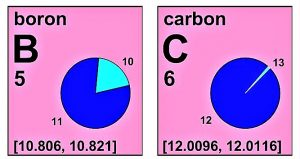Themes

# What is the atomic weight?

## Atomic weight

The atomic weight comprises all that dimensionless physical quantity which is understood as the ratio of all the masses that comprise the atoms within an element.## What is the atomic weight?

Modern chemistry establishes the atomic weight as the average value of the weight of the isotopes that make up a chemical element . This physical value is dimensionless and is associated with each chemical element present within the periodic table. It is also known as Relative Atomic Mass.

• Definition of atomic weight
• How it is calculated
• What is atomic weight for
• How it differs from atomic mass
• Importance
• Examples of atomic weight

## Definition of atomic weight

The IUPAC defines it as the ratio of the average mass of the atoms of a chemical element with respect to one twelfth of the mass of a Carbon-12 atom.

## How it is calculated

To mathematically measure the corresponding value of an atomic weight, the value of the atomic mass of an isotope of the chemical element studied must first be calculated . This mass corresponds to the number of protons and neutrons that this isotope contains. Then, the quotient between the atomic mass of an isotope of the element and one twelfth of the mass of a Carbon-12 atom must be calculated . In this way, the atomic weight relative to said isotope is calculated. This calculation must be done for each existing isotope of the chemical element.

Subsequently, the value of the atomic weight relative to each isotope must be multiplied by its percentage relative abundance, calculate the sum of these values ​​and, finally, divide by 100 to know the atomic weight relative to the chemical element.

## What is atomic weight for

Its usefulness lies in that it allows the quantified measurement of the components and / or substances present within a chemical reaction through their relationship with the molar mass , which is the mass of one mole of said molecule . That is to say, it allows calculating the amount of matter present in a given substance, which is of utmost importance in the study of chemical reactions.

## How it differs from atomic mass

The difference is that the atomic weight is a dimensionless measure that starts from the ratio between the average mass of the atom of a chemical element and one twelfth of the isotope of carbon-12 . Instead, atomic mass is a dimensional measure that corresponds to the average amount of matter present in a chemical element atom.

## Importance

The emergence of this concept allowed the successive development of chemistry as a fundamental science and the deepening of technological innovations in this area . By facilitating the calculation of the amount of mass present within a substance through the molar mass, the way was opened for the birth of analytical chemistry, being of vital importance for our modern world.

## Examples of atomic weight

• Atomic weight of iron (Fe): 55.845 u
• Sulfur (S): 32,065 u
• Sodium (Na): 22.989769 u
• Nitrogen (N): 14.0067 u
• Chlorine (Cl): 35.453 u
• Atomic weight of aluminum (Al): 26.981539 u
• Hydrogen (H): 1.00794 u
• Chromium (Cr): 51.9961 u
• Of the cobalt (Co): 58.933195 u
• Argon (Ar): 39.948 u
• Titanium (Ti): 47.867 u
• Silicon (Si): 28.0855 u
• Atomic weight of oxygen (O): 15,999 u
• Boron (B): 10,811 u
• Calcium (Ca): 40.078 u
• Magnesium (Mg): 24.305 u
• Potassium (K): 22.989769 u
• Carbon (C): 12.0107 u
• Helium (He): 4.002602 u
• Of the molybdenum (Mo): 95.94 u
• Tantalum (Ta): 180.94788 u
• Atomic weight of vanadium (V): 50.9415 u
• From niobium (Nb): 92.90638 u
• Fluorine (F): 18.998403 u
• Neon (Ne): 20.1797 u
• Uranium (U): 238.02891 u
• Tin (Sn): 118.71 u
• Zinc (Zn): 65.38 u
• Barium (Ba): 137.327 u
• Atomic Weight of Gold (Au): 196.96657 u
• Del bomo (Br): 79.904 u
• Cesium (Cs): 132.90545 u
• Phosphorus (P): 30.973762 u
• Lead (Pb): 207.2 u
• Atomic Weight of Silver (Ag): 107.8682 u

Check Also
Close